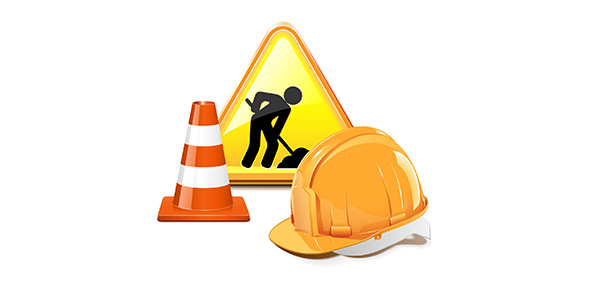# Construction

15 Questions | Total Attempts: 263SettingsTime: 30 Minute

Related Topics
• 1.
With the help of a ruler and a compass, it is possible to construct an angle of:
• A.

50o

• B.

40o

• C.

37.5o

• D.

29o

• 2.
In the construction of ΔABC, AB = 5 cm, ∠A=75o is not possible when difference of BC and AC is equal to:
• A.

3.5 cm

• B.

4 cm

• C.

4.5 cm

• D.

5.5 cm

• 3.
In the construction of ΔABC, BC = 6 cm, ∠B = 45° is not possible when difference of AB and AC is equal to:
• A.

6.9 cm

• B.

5.2 cm

• C.

5 cm

• D.

4 cm

• 4.
Which of the following angles cannot be constructed with the help of a ruler and a compass?
• A.
• B.
• C.
• D.
• 5.
Two or more circles are congruent if they have same:
• A.

• B.

Diameter

• C.

• D.

None of the above

• 6.
A quadrilateral could be drawn, if the measures of its:
• A.

Four angles and one side are given

• B.

Four sides are given

• C.

Three sides and a diagonal are given

• D.

Four sides and one angle are given

• 7.
The point in the plane of a triangle which is at equal perpendicular distances from the three sides of the triangle is its:
• A.

Incentre

• B.

Circumcentre

• C.

Orthocentre

• D.

Centroid

• 8.
On a ray AB with initial point A, taking A as centre and some radius, draw an arc of a circle, which intersects AB, say at a point D. Taking D as centre and with the same radius as before, draw an arc intersecting the previously drawn arc, say at a point E. Draw the ray AC passing through E. Then, the measure of ∠CAB is
• A.

15o

• B.

30o

• C.

45o

• D.

60o

• 9.
It is possible to construct a triangle ABC whose sides are:
• A.

2 cm, 3 cm and 5 cm

• B.

3 cm, 2 cm and 6 cm

• C.

3 cm, 4 cm and 5 cm

• D.

4 cm, 3 cm and 8 cm

• 10.
To construct a right triangle whose base is 12 cm and the sum of its hypotenuse and the other side is 18 cm. We draw a line segment AB of 12 cm. Draw a ray AX making 90o with AB. The next step is:
• A.

Cut a line segment BD 18 cm on AX

• B.

Cut a line segment AD 18 cm on AX

• C.

Cut a line segment AD 18 cm on AB

• D.

Cut a line segment BD 18 cm on AB

• 11.
A triangle divides the plane into____ parts.
• A.

2

• B.

3

• C.

4

• D.

6

• 12.
In ΔABC if ∠B =∠C=30o, which of the following is the longest side?
• A.

AC

• B.

BC

• C.

AB

• D.

None of the above

• 13.
In a ΔABC if ∠A=50o and ∠B=70o then the shortest and the largest sides of the triangles are:
• A.

BC, AC

• B.

AB, BC

• C.

AB, AC

• D.

None of the above

• 14.
What is the supplementary angle of 108o ?
• A.

70.5o

• B.

71o

• C.

72o

• D.

72.5o

• 15.
In the construction of ΔABC, BC=3 cm, ∠C=600, is possible when difference of AB and AC is equal to:
• A.

2.8 cm

• B.

3 cm

• C.

3.1 cm

• D.

3.2 cm